Created November 19, 2022 © MIT

# 2-axis Camera Controller using PSoC4100

I'll be building a camera controller - servo mounted using the PSoC 4100 pioneer kit.

IntermediateFull instructions provided2 hours7

## Things used in this project

### Hardware components

 Infineon PSoC™ 4100S Capsense Pioneer Kit
×1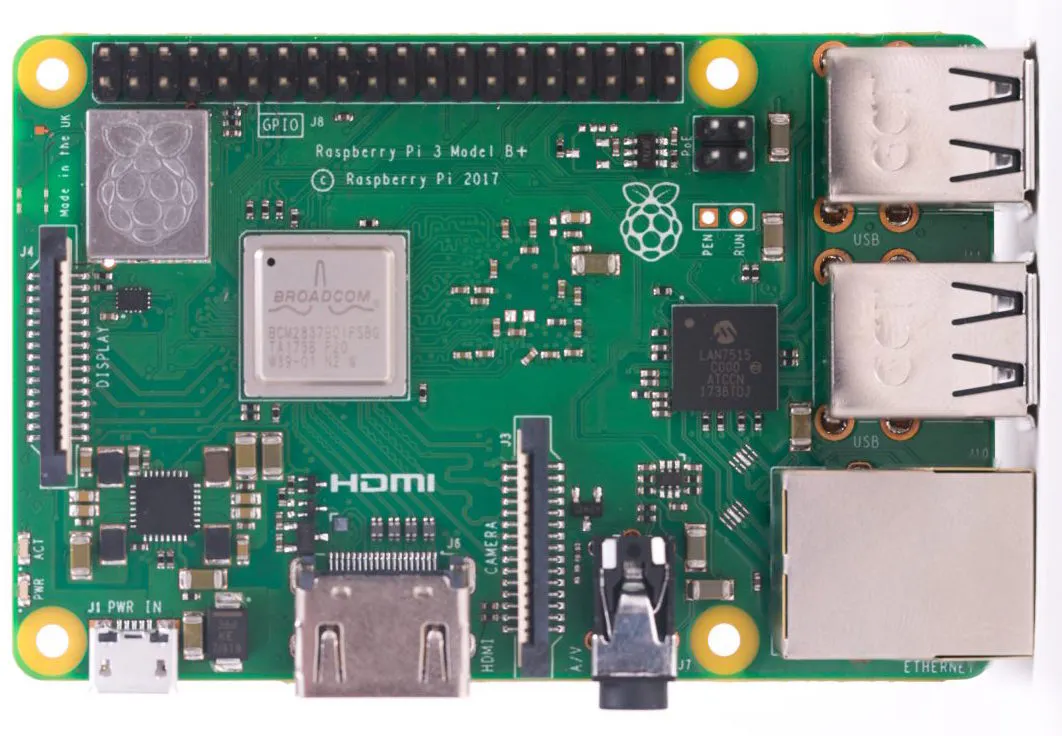Raspberry Pi 3 Model B+
×1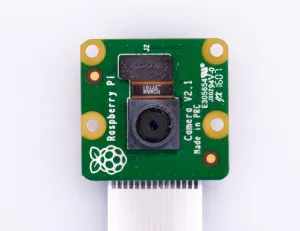Raspberry Pi Camera Module V2
×1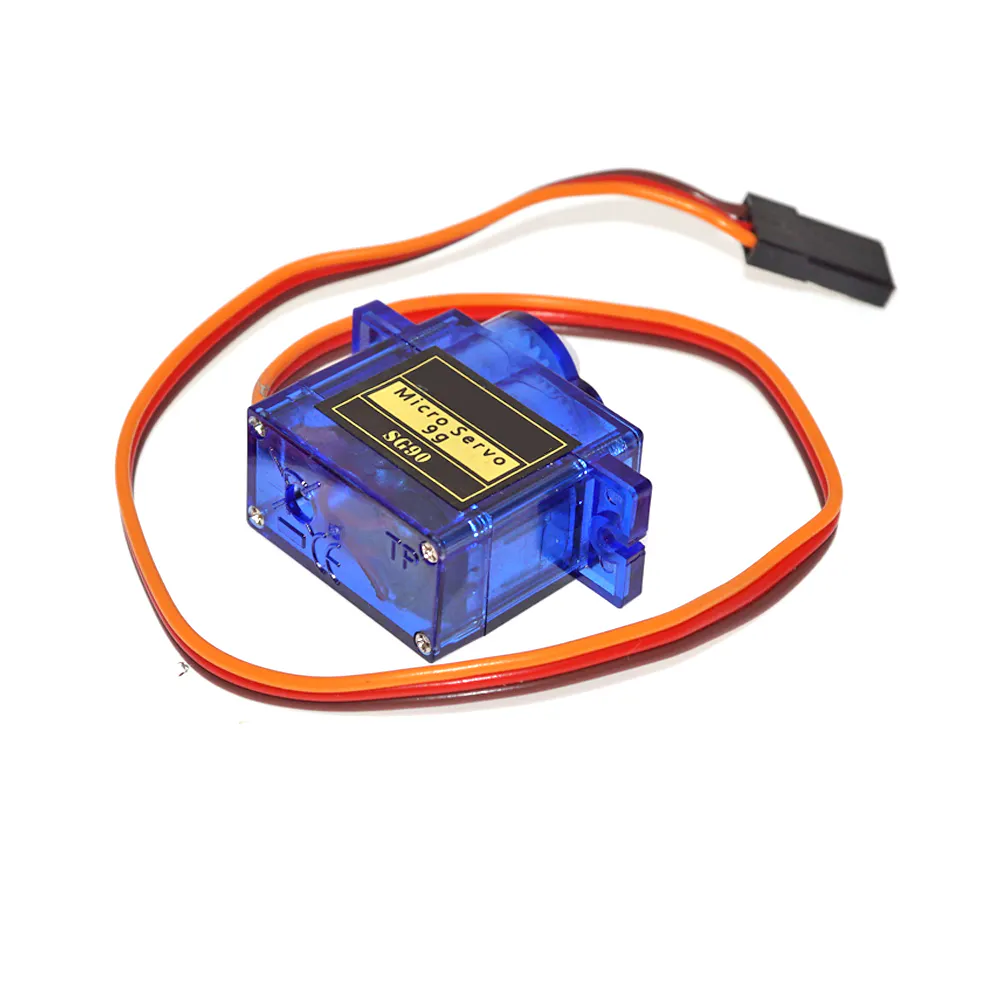SG90 Micro-servo motor
×2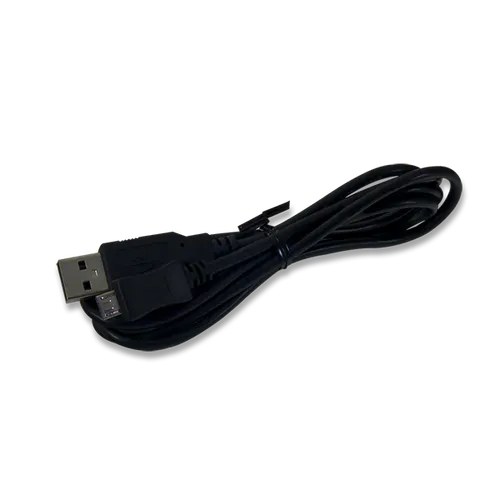Digilent USB A to Micro-B Cable
×1

### Software apps and online services

 Raspbian OS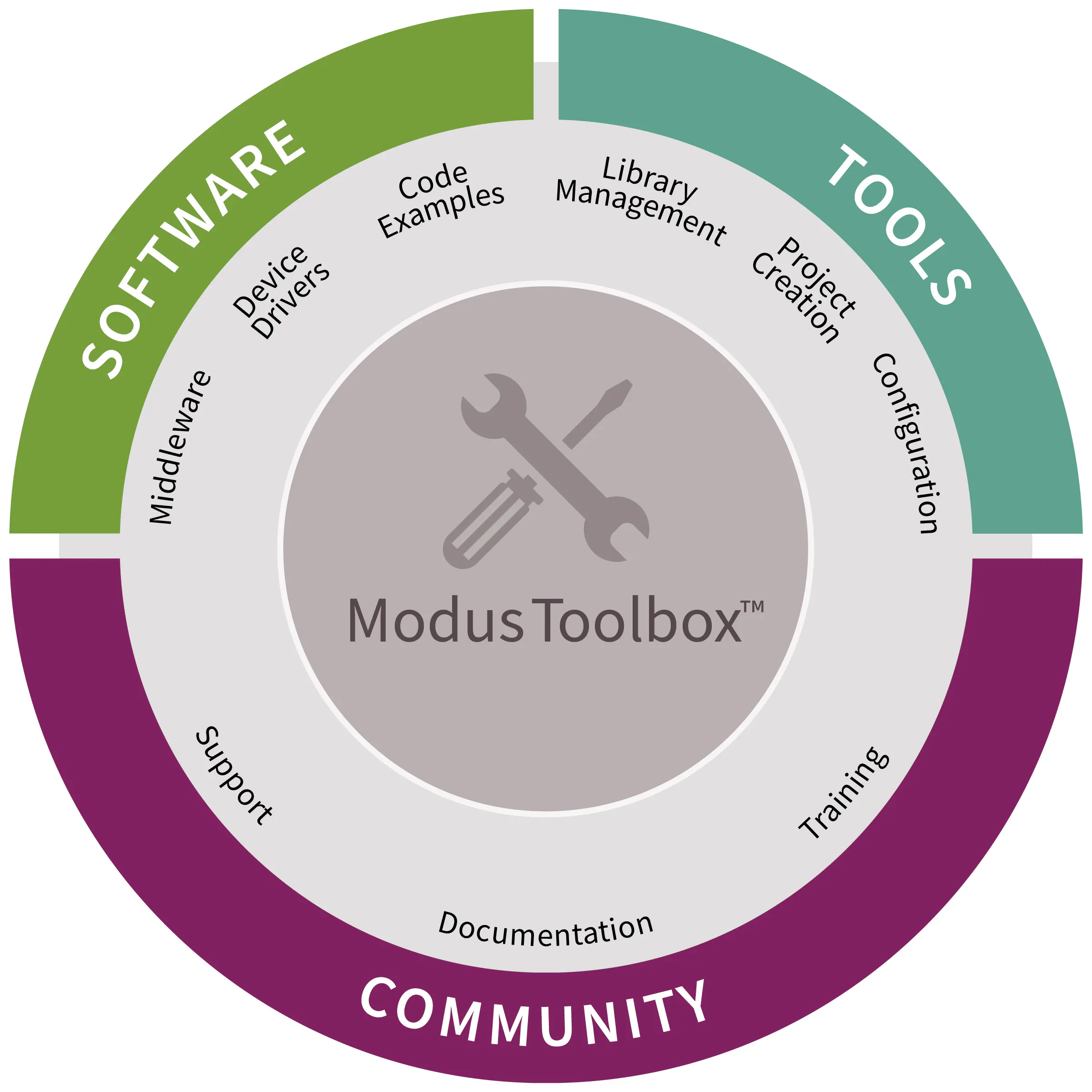Infineon ModusToolbox™ Software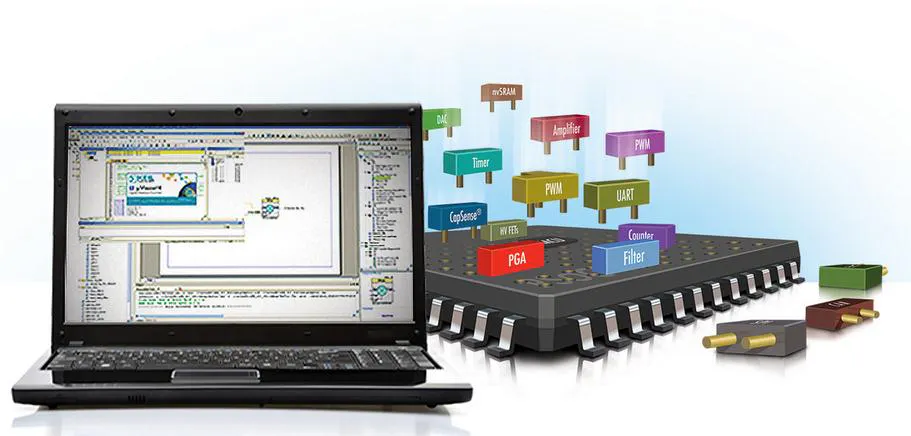Cypress PSoC Creator

### Hand tools and fabrication machines3D Printer (generic)

## Schematics

### components_raspi_pca9685_i2c_with_servo_RUwUiZhD0U.jpg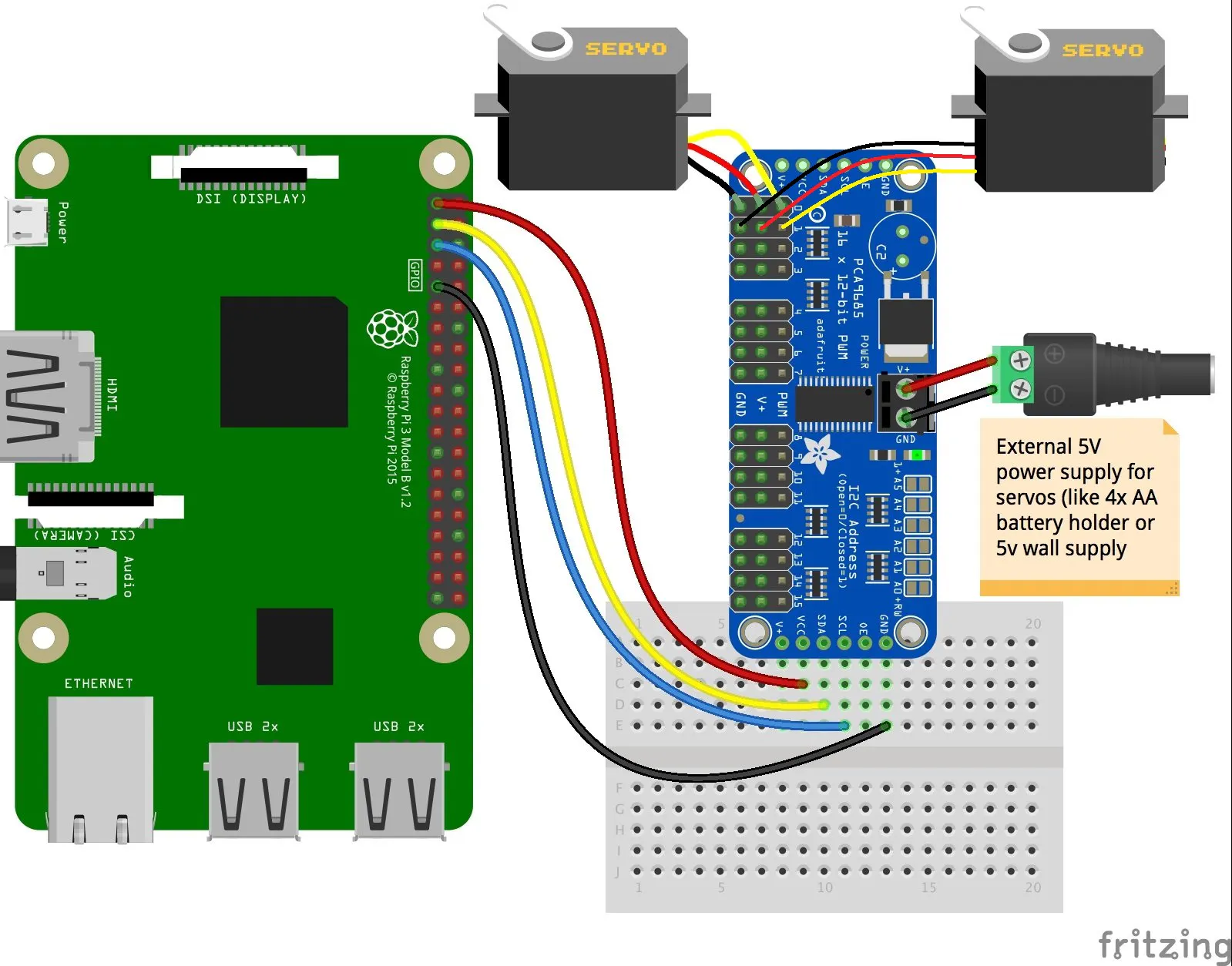## Code

### Capsense_camera_controller.py

Python
```from pynput.mouse import Listener
import time
from picamera import PiCamera
from time import sleep
camera = PiCamera()
camera.rotation = 0

kit = ServoKit(channels=16)

prevx = 0
prevy = 0

kit.servo.angle = 0
kit.servo.angle = 0

angle0 = 0
angle1= 0

camera.start_preview()

def on_move(x, y):
global prevx, prevy, angle0, angle1

#print('Mouse moved to ({0}, {1})'.format(x, y))
#print('prev moved to ({0}, {1})'.format(prevx, prevy))

print('servo0 {0}'.format(angle0))

if((prevx-x)>0) and angle0 >= 0 and angle0 <= 180:
kit.servo.angle = angle0
if(angle0 < 180):
angle0 = angle0 + 10
print('servo0 + {0}'.format(angle0))

elif((prevx-x)<0) and angle0 >= 0 and angle0 <= 180:

kit.servo.angle = angle0
if(angle0 > 0):
angle0 = angle0 - 10
print('servo0 - {0}'.format(angle0))

if((prevy-y)<0) and angle1 >= 0 and angle1 <= 180:
kit.servo.angle = angle1
if(angle1 < 180):
angle1 = angle1 + 10
print('servo1 + {0}'.format(angle1))

elif((prevy-y)>0) and angle1 >= 0 and angle1 <= 180:
kit.servo.angle = angle1
if(angle1 > 0):
angle1 = angle1 - 10
print('servo1 - {0}'.format(angle1))

prevx = x
prevy = y

def on_click(x, y, button, pressed):
pass

def on_scroll(x, y, dx, dy):
pass

with Listener(on_move=on_move, on_click=on_click, on_scroll=on_scroll) as listener:
listener.join()

```

## Credits

### Rahul Khanna D

40 projects • 205 followers
Research Enthusiast - Computer Vision, Machine Intelligence | Embedded System | Robotics | IoT | Intel® Edge AI Scholar